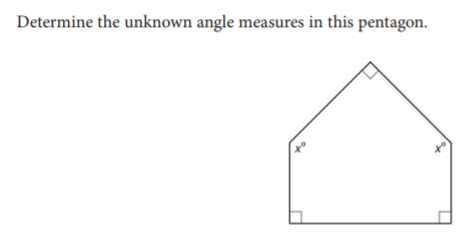# Mod 7.1 Interior and Exterior Angles

Topic:
Angles

## Triangle Sum Theorem

Change the angles of the above triangle. What is the sum of all three interior angles of the triangle?

When will the sum of the interior angles of a triangle add up to 180 degrees?

Is it possible to create a triangle that the interior angles do not add up to 180 degrees?

## Notice when the sides of the angles are adjacent and the vertices meet at one point, they form a straight angle.

What is the measure of a straight angle?

## Take Note

Triangle Sum Theorem: The sum of the angle measures of a triangle is 180 degrees.

## Take Note:

Polygon angle sum theorem: The sum of the measures of the interior angles of a convex polygon with n sides is (n-2)180o

## Polygon Angle Sum

Using the slides on the above image, determine the sum of the interior angles of a dodecagon (12 sided polygon).

Using the slides on the above image, determine the sum of the interior angles of a hexagon (6 sided polygon).

Using the slides on the above image determine the sum of the interior angles of a pentagon (5 sided polygon)To determine the unknown angles of this pentagon we need to first determine the sum of the interior angles of a pentagon using the polygon angle sum theorem. (n-2)180o We determined that measure in the previous question and found the sum of the interior angles of a pentagon is 540o Write an equation adding up all interior angles and set it equal to 540o Solve for xo

What is the measure of angle x in the pentagon above?

## Looking at image above

What is the ? Set up the equation to solve for x. Solve for x, then substitute that value for x into the equation to determine the measure of angle B.

## Solve the following.

What is the ?

What is the unknown angle measure xo?

What is the from above?

What is the measure of the two remote interior angles from above?